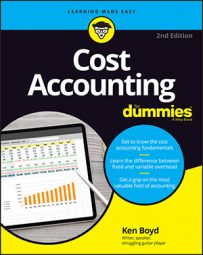##### Cost Accounting For DummiesThe degree of operating leverage is a cost accounting formula that shows how well you’re using your fixed costs to generate a profit. The more profit you can generate from the same amount of fixed cost, the higher your degree of operating leverage.

Here’s the formula:

Degree of operating leverage = contribution margin ÷ profit
Profit = contribution margin – fixed costs

First, calculate the contribution margin. (Assume your product, a software application, sells for \$40 per unit and your variable costs are \$20 per unit. Use the number of units from the target net income discussion above:

Contribution margin = sales – variable costs
Contribution margin = \$40 x (150 units) – \$20 x (150 units)
Contribution margin = \$20 x (150 units)
Contribution margin = \$3,000

Use the degree of operating leverage formula to compute the degree of operating leverage:

Degree of operating leverage = contribution margin ÷ (contribution margin – fixed costs)
Degree of operating leverage = \$3,000 ÷ (\$3,000 – \$1,000)
Degree of operating leverage = \$3,000 ÷ \$2,000
Degree of operating leverage = 1.5

Degree of operating leverage can also be defined as contribution margin divided by profit. It’s saying the same thing. All the calculations simply amount to this statement: “At sales of 150 units, contribution margin is 1.5 times profit.”

You also can use the degree of operating leverage formula to assess the relationship between costs and profit. If you minimize your fixed costs, you increase your profit. Even better, you can earn more profit without changing your sale price, contribution margin, or units sold.

Here is how the degree of operating leverage looks if fixed costs were only \$500:

Degree of operating leverage = \$3,000 ÷ (\$3,000 – \$500)
Degree of operating leverage = \$3,000 ÷ \$2,500
Degree of operating leverage = 1.2

The ratio went down from 1.5 to 1.2. Now contribution margin (\$3,000) is 1.2 times profit (\$2,500). Contribution margin did not change. Because fixed costs went down, profit increased.

What if fixed costs went down to zero? Contribution margin/profit would be 1, and that’s a trophy position! Your entire contribution margin goes toward profit.